# Probability outcomes for coins, dice, and spinners#### All in One Place

Everything you need for better grades in university, high school and elementary.#### Learn with Ease

Made in Canada with help for all provincial curriculums, so you can study in confidence.#### Instant and Unlimited Help

0/2
##### Intros
###### Lessons
1. Introduction to Probability Outcomes for Coins, Dice, and Spinners:
2. What is probability?
3. What are outcomes in probability?
1. Outcomes for flipping a coin
2. Outcomes for rolling a 6-sided die
3. Outcomes for a spinner with different regions
0/7
##### Examples
###### Lessons
1. Outcomes are the result of flipping coins, rolling dice, or turning a spinner's arrow
List all the possible outcomes. How many outcomes are there in total?
1. A coin with a happy face or sad face on its sides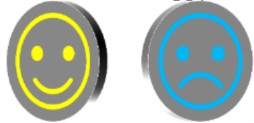2. An 8-sided die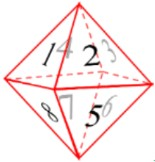3. Two friends having a race against each other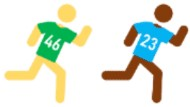2. Outcomes that are equally as likely to occur
Fill in the table for the probability outcomes
1. Rolling a 6-sided die: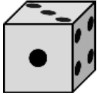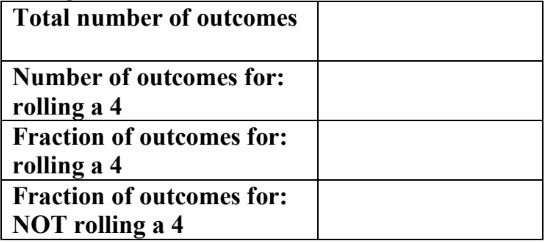2. Turning a spinner with letters: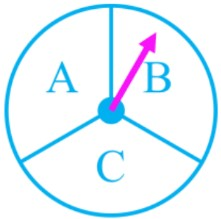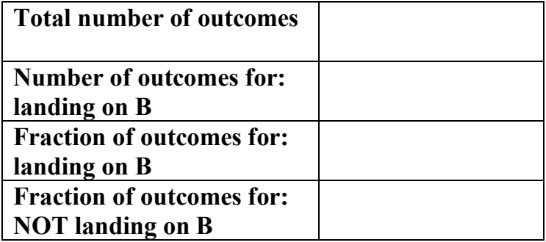3. Turning a spinner with numbers: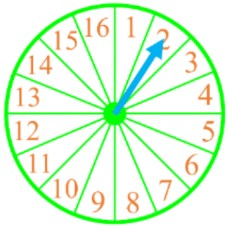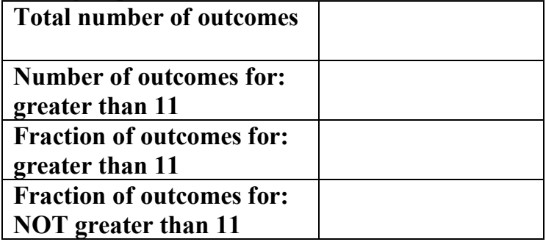4. Rolling a 6-sided die: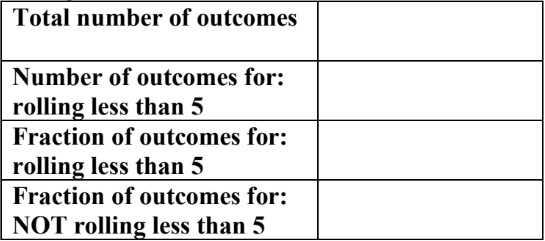###### Topic Notes

In this lesson, we will learn:

• What is probability in math?
• How to write probability as a fraction
• How to list all the outcomes for probability events (using coins, dice, and spinners)

Notes:

• Probability is math for events that only sometimes happen. The chances of something happening can be likely or unlikely to happen.
• Calculating probability is like predicting the future. We are trying to get a measure of the chances that something will happen.

• Arithmetic math (all basic operations; adding, subtracting, multiplying, dividing numbers) is certain. In comparison, probability math is uncertain--but still predictable.

• Probability can be given as a fraction, following the formula format:

• Probability = $\frac{number\,of\, outcomes\, wanted} {total\,number\,possible\,outcomes}$

• Outcomes are all the possible endings that could happen for a situation.
• Some simple probability situations that are often used in math problems include: tossing a coin, rolling a six-sided die, and spinning the arrow on a spinner.

• Coins: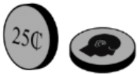• If you toss a coin, it will land on a flat side—either on heads or tails
• So, there are 2 possible outcomes to a coin toss: heads or tails
• The probability of landing on heads is $P$(heads) = $\frac{1}{2}$
• The probability of landing on heads is $P$(tails) = $\frac{1}{2}$

• Dice: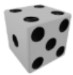(a six-sided die, if singular)
• When rolling a die, it will land with one of its six flat faces facing up (on top). There are six sides labelled from 1 to 6.
• So, there are 6 possible outcomes to a die roll: 1, 2, 3, 4, 5, or 6
• The probability of landing on any one side (1-6) is one out of six chances
• $P$ (1) =$P$ (2) = $P$ (3) = $P$ (4) = $P$ (5) = $P$ (6) = $\frac{1}{6}$

• Spinners: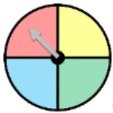(can have any number of equal parts; labels with numbers, letters, etc.)
• When using a spinner, the arrow will land on one of the marked regions. In this case, there are 4 different coloured regions.
• So, there are 4 possible outcomes for this spinner: red, yellow, green, or blue
• The probability of landing on any one of the colors is one out of four chances
• $P$ (Red) = $P$ (Yellow) = $P$ (Green) = $P$ (Blue) = $\frac{1}{4}$